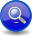#Boost C++ Libraries

...one of the most highly regarded and expertly designed C++ library projects in the world.

##### Sequence (```a >> b```)
###### Description

The sequence operator, `a >> b`, parses two or more operands (`a`, `b`, ... etc.), in sequence:

```a >> b >> ...
```
```// forwards to <boost/spirit/home/qi/operator/sequence.hpp>
#include <boost/spirit/include/qi_sequence.hpp>
```

Also, see Include Structure.

###### Model of

Notation

`a`, `b`
###### Expression Semantics

Semantics of an expression is defined only where it differs from, or is not defined in `NaryParser`.

Expression

Semantics

```a >> b```

Match `a` followed by `b`.

###### Attributes

Expression

Attribute

```a >> b```

```a: A, b: B --> (a >> b): tuple<A, B>
a: A, b: Unused --> (a >> b): A
a: Unused, b: B --> (a >> b): B
a: Unused, b: Unused --> (a >> b): Unused

a: A, b: A --> (a >> b): vector<A>
a: vector<A>, b: A --> (a >> b): vector<A>
a: A, b: vector<A> --> (a >> b): vector<A>
a: vector<A>, b: vector<A> --> (a >> b): vector<A>```

###### Complexity

The overall complexity of the sequence parser is defined by the sum of the complexities of its elements. The complexity of the sequence itself is O(N), where N is the number of elements in the sequence.

###### Example

Some using declarations:

```using boost::spirit::ascii::char_;
using boost::spirit::qi::_1;
using boost::spirit::qi::_2;
namespace bf = boost::fusion;
```

NoteThe test harness for the example(s) below is presented in the Basics Examples section.

Simple usage:

`test_parser("xy", char_ >> char_);`

Extracting the attribute tuple (using Boost.Fusion):

```bf::vector<char, char> attr;
test_parser_attr("xy", char_ >> char_, attr);
std::cout << bf::at_c<0>(attr) << ',' << bf::at_c<1>(attr) << std::endl;```

Extracting the attribute vector (using STL):

```std::vector<char> vec;
test_parser_attr("xy", char_ >> char_, vec);
std::cout << vec << ',' << vec << std::endl;```

Extracting the attributes using Semantic Actions (using Phoenix):

```test_parser("xy", (char_ >> char_)[std::cout << _1 << ',' << _2 << std::endl]);
```